• 线性代数入门指南：线性方程组
千次阅读
2019-12-07 21:52:43

一、线性方程组

如果说用从高等数学的视角和从初等数学的视角看待线性方程组有什么差异的话，我要说，高等数学为我们提供了全新的理解线性方程组的角度。对于任意一个线性方程组，不同于高中单纯的视角，我们有这样几种理解这个方程组的角度。第一是将这个线性方程组理解成一个向量方程组，第二个是将这个方程组以矩阵乘法AX=B的形式写出来。对于第二种，B是一个n阶列向量，如果这个向量是零向量，那么方程组是齐次的，反之则是非齐次的。于是从这两个角度出发我们能得到一些新的结论。

第一，线性方程组有解不再只是线性方程组有解，还可以理解为B可以被A中n个列向量线性表示。还有一种理解方式是向量组a1,a2 … …an和a1,a2 … …an,B是等价的，这意味着B必然是向量组张成空间当中的一个向量，而这恰恰说明了一个事实——系数矩阵和增广矩阵的秩是相同的（毕竟向量空间的维数是一样的）

如果B不是前一个向量组张成空间的向量呢？那么很遗憾，这个线性方程组无解。这个无解的最为明显的体现就是在对增广矩阵进行初等变换到最后，也许会出现类似于0=1这样无厘头的式子，这可真让人无奈呀。

第二，线性方程组的解可以写成向量的形式，这便是解向量，得出这个结论是基于矩阵的视角看待线性方程组。

在初等数学的背景下，我们一直在一种“理想”的环境当中解线性方程组，不仅未知数的个数较少，而且往往只有唯一解，然而如果沉醉其中，我们便无法站在高等数学的山峰上仰望星空，并在残酷的现实当中撞得头破血流。

二、齐次和非齐次线性方程组的解

理想情况下，对于一个线性方程组，有n个未知数和n个方程，而且方程右边的系数还不都是0，这样我们可以得到方程的唯一解，这也是我们在学习初等数学时的工作。

这种情况用现在学过的定理描述就是，对于一个非齐次线性方程组，在系数矩阵的秩和增广矩阵的秩相同，并且等于未知数个数的情况下，我们的方程组有唯一的解。

那么，问题来了。

我们不妨看看这条定理的限定词，“非齐次”“秩相同”“等于未知数个数”，这三个条件满足才能得到唯一解，也就是说破坏了其中任意一个的话结果就大径相庭。比如说，我们把这个“等于未知数个数”改成了“不等于未知数个数”，那这个结果会是什么样的呢？如果比未知数多，那么很可能这些方程当中有重复的，或者还可能有一些方程自相矛盾（这就没辙了）；如果比未知数少，这样的话就有一些未知数失去了方程的限定，可以放飞自我了。（对，它们就是自由未知数）这样诞生了无穷多个解。再比如，如果把“非齐次”改成了“齐次”，这又是什么情况呢？这是一种很没有意思的情况，它意味着如果把方程组通过加减消元法消消消消消，得出来的结果就是一排的鸡蛋——0。

总结一下，秩是否和未知数个数相等，决定了一个方程是否有无数个解。而对于齐次的线性方程组，如果有唯一解，那么只能是0。

以上只是纯粹基于线性方程组的角度分析线性方程组的解的问题。还记得写在开头的那句话吗？看待线性方程组的问题不只有线性方程组本身这个角度，还可以从矩阵的角度去分析。

由于线性方程组还可以写成是矩阵的形式，即AX=B，而矩阵可以理解为是“线性变换后空间的各个基向量坐标”，X和B恰好又是一个个向量。这意味着什么呢？我们知道每一个矩阵都对应着一种线性变换，而矩阵的乘法则是行向量与列向量做内积运算，内积从几何上看是一个向量在另一个向量上投影的长度和另一个向量长度的乘积。因此，AX=β的含义，还可以理解为“X向量在A作为基向量的坐标系下应该写成β”，或者“X向量在经历了A矩阵所描绘的线性变换后变成了β向量”。

明白了这一点之后，由于不同的矩阵对应的线性变换的效果不同，我们不妨把AX=B的情况依据“A是否是方阵”和“B是否为0”两类讨论。我们知道，如果一个矩阵是满秩的方阵，那么它对空间的作用效果只有剪切和旋转，并不会改变空间的维度。而如果是不满秩的方阵，则对空间有降维的效果。所以，对于AX=B，如果A是满秩的，意味着一个空间当中的向量在经过A的变换后不会改变维数，如果B是零向量，只能说明一开始就是零向量，即X=0，线性方程组是零解；如果B是特定的一个向量，那一定存在一个唯一的X；如果是不满秩的方阵，那么这个X向量一定是被降维了，而降维意味着信息丢失，无法逆推（这也就是为什么不满秩的矩阵没有逆矩阵），所以X无从猜测，有无数种可能——线性方程组有无穷解。

那么，如果A不是方阵呢？我们看一个比较简单的例子吧。

x + 2y + z = 1
x + y + 2z = 0

我们用矩阵的方式重新书写这个方程就会发现，[ x , y , z]’ 这个向量最终变成了 [1,0]，这似乎在说A这个不是方阵的2×3矩阵有降维的功能，这是什么原因呢？我想有两种解释，第一是因为这个矩阵秩最多为2，也就是说矩阵的列向量只能线性表示二维的向量，所以必然有一个维度的信息丢失了。第二种解释其实和第一种殊途同归，我们知道矩阵乘法是向量之间做内积的过程，AX=B是A的行向量和B的列向量做内积，而A只有两个行向量呀！所以最后的结果是一个二维的向量难道不是很自然的事情吗？

事实上，我们对问题的划分标准“A是否为方阵”“方阵A是否满秩”共同指向了对一个事实的询问——向量X是否被降维了？如果被降维了，那么这个线性方程组必然有无数个解，反之则有唯一解：零向量变换后仍然是零向量，非零向量变换后依旧是非零向量，就像你爹十年后仍然是你爹，儿子永远不会变成爸爸。

再思考一下，在这种情景下，解空间意味着什么呢？为了回答这个问题，我们不妨先从AX=0这个齐次线性方程入手。从线性变换的观点来看，这个方程表明X向量经过A的变换变成了一个零向量，于是答案呼之欲出。对于AX=0的解空间，我们能知道它是所有在线性变换当中变成了零向量的向量的集合。这个集合又被称为矩阵A的零空间，或者矩阵A的核。

非齐次的线性方程组的通解可以看成是对应的齐次线性方程组的解加上一个特解。对于这个特解，我们可以理解为某一个向量在A代表的线性变换当中被降维成了β。但实际上特解并不是唯一的，因为还有其他诸多向量在线性变换当中被降维成了零向量，从这些向量中随意抽出一个放在特解上又是一个新的特解。所以，综上所述，解空间是一切在线性变换当中被降维的向量的可能性。

感性地看待数学，把向量比作人的话，生活就像降维的矩阵，“萍水相逢，尽是他乡之客”，人与人未曾相识，各有不同，却在同一时刻同一地点相逢。好在这个类比并不完全正确，“往者不可谏，来者犹可追”，无论我们拥有怎样的过去，我们仍然拥有明天。

三、cramer法则及其几何原理

记得有个关于程序猿的段子是这么说的：困扰程序猿的问题通常有两种，一种是为什么这段程序不能运行，另一种是为什么这段程序能运行。

人类科学发展史上似乎常常出现这种现象：在彻底弄清一种技术的原理之前，技术先被投入应用了，就比如量子力学当中经典的普朗克方程，他的提出者在提出这个公式时甚至无法清楚地解释其中的原理，只是用数学方法将两个公式统一了起来。很巧的是，行列式最早被发明出来只是作为解线性方程组的一个数学工具，囿于时代限制，孤立于数学体系之外，直到范德蒙和柯西出现，行列式的潜在价值才得到了开发。

单纯地从抽象的代数证明上弄清cramer 法则的原理未免有些无趣。倘若我们从向量的视角观察行列式的话，我们还能从几何上理解为什么cramer法则能以一种奇妙的方式得出线性方程组的解。从一个简单的例子开始吧。

以一个二阶行列式为例（假设它不为0），我们知道它的几何意义是表示列向量围成的一块平行四边形的面积。好吧，假设一个二元一次方程组的行列式可以写成 |α1 α2| ，依据cramer法则我们知道，如果想求出x，我们需要一个行列式叫做：| β α2 |

这两个行列式有什么联系吗？它们共同表示了一个平行四边形的面积，而且还是同底的平行四边形的面积。接着我们需要用到一点点线性方程组的知识和行列式的性质。

首先，这个方程组可以用向量方程的形式表示为：x α1 + y α2 = β接着借助行列式的性质，| β α2 | 实际上能写成 | xα1 α2 | 。于是我们明白了，| β α2| 和 | α1 α2 |的比值就是我们梦寐以求的x！在几何上，这就是一个同底的平行四边形的面积比。

这个思路推广到三阶行列式的话并不会遇到任何困难，同底的平行四边形的面积比变成了同底面积的平行六面体的体积比。实际上这个思路对n阶行列式仍然适用。

有一种情况是无法使用cramer法则的，那就是当系数行列式为0时。单纯地理解方式就是分母不能为0。从几何上考虑的话，行列式为0意味着空间在线性变换后被降维，就像三维空间被打成了二维，此时讨论“体积比”不存在任何意义，因为根本不存在体积。

四、线性和方程

最初学线性方程组时，我犯了惯性思维的毛病，把这里提到的线性方程理解成了简简单单的ax+by=c这样的形式，然而这种观念大概是中世纪的数学观念，当数学在近代蓬勃发展时，“方程”已经不仅仅是我们所认识的那个稚嫩的概念了。

“含有未知数的等式就是方程”，这是我印象当中中学课本上的定义。但站在更高的角度去看待方程，我理解的方程是具有四个要素的：未知量，已知量，运算法则，等号。这里的未知量和已知量已经不单单是一个常数了，它还可以是一个函数。

理解了方程，我们再思考一下“线性”，“线性”这个概念对于线性代数的重要性不亚于加减乘除在初等数学当中的重要性，因为它代表了线性代数的研究对象的运算法则。在数学上，线性意味着两个性质：叠加和齐次。

T（a）+T（b）=T（a+b）
T（ka）=kT（a）

于是我们很容易发现，线性代数的研究对象——向量，正是满足这样的法则，在几何上，几乎也是很直观地呈现了“线性”。然而线性的运算并不是只有向量的加法和数乘，极限也好，微分也罢，这些运算法则同样符合线性。这意味着，我们所认为的线性方程远比我们想象的要广阔。比如线性微分方程，它仍然是线性的方程，自然而然会呈现出和上述讨论内容相似的性质。线性微分方程中既有线性无关、线性相关的概念，又有特解和基础解组的概念，原因大抵如此吧。入门
更多相关内容
• 本文实例讲述了Python线性方程组求解运算。分享给大家供大家参考，具体如下： 求解线性方程组比较简单，只需要用到一个函数(scipy.linalg.solve)就可以了。比如我们要求以下方程的解，这是一个非齐次线性方程组： 3x...
• 1.描述：求解线性方程组Ax=b，写成函数。其中，A为n乘n阶矩阵，x为n元未知向量，b为n个常数组成的矩阵。  2.要求： 采用高斯先列主元消元法（也可采用其他方法）求解线性方程组AX=b。 鼓励可视化编程；   源...
• 在MVG(多视图几何)和机器学习领域，求解线性方程组几乎是所有算法的根本，本文旨在帮助读者搞懂矩阵分解与线性方程组的关系，并给出利用SVD求解线性方程组的实战代码。 本资源是博文"【动手学MVG】矩阵分解与线性...
• 为求解一个线性方程组，首先构造增广矩阵[A|B]，采用偏序选主元策略的高斯消去法变换成上三角矩阵，再执行回代过程得到解。 输入形式 在屏幕上依次输入方阵阶数n，系数矩阵A和常数矩阵B。 输出形式 首先输出上三角...
• 程序里可实现对矩阵A进行LDU分解，通过LDU分解、前代、规格化、回代四个步骤求解线性方程组Ax=b。
• 雅可比迭代 使用雅可比迭代法求解线性方程组的步骤 步骤1输入系数矩阵A和方程组右端向量B 步骤2将矩阵A分解为下三角阵L对角阵D和上三角阵U 可分解为D+L+UX=B for o=1:n d(o,o)=a(o,o; u(o,o+1:n)=-a(o,o+1:n; end ...
• 讲述了矩阵方程求解的定常迭代算法 - 经典(定常,不动点)迭代法: Jacobi/Gauss-Seidel,SOR,AOR等 - Krylov子空间迭代法: CG,MIRES,GMRES,BiCGStab等
• 线性方程组的求法与示例详解 线性方程组 由n个1维未知量，m个方程组成的组合叫做线性方程组。 特别的当方程组右边的值全都是0时叫做齐次线性方程组。 增广矩阵 在系数矩阵的右边添上一列，该列由线性方程组...

线性方程组的求法与示例详解

# 线性方程组

由n个1维未知量，m个方程组成的组合叫做线性方程组。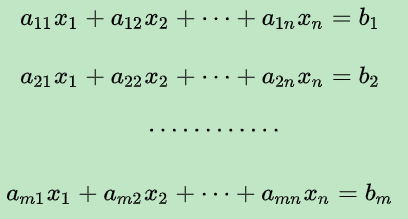特别的当方程组右边的值全都是0时叫做齐次线性方程组。

# 增广矩阵

在系数矩阵的右边添上一列，该列由线性方程组等号右边的值按照顺序拼接而成，该新的矩阵叫做方程组的增广矩阵。针对如下线性方程组，我们不难得到其系数矩阵（即由每个未知量前的系数按照顺序组成的矩阵）是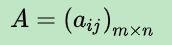而我们假设一列(方程组右边的值)构成新的矩阵即叫做该方程组的增广矩阵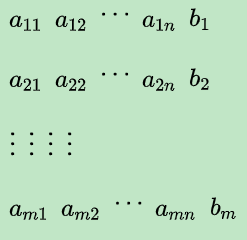或者更一般的，如果我们把线性方程组简写为Ax=b那么增广矩阵B可以记作(A,b)。

# 矩阵的秩

设在m×n的矩阵A中有一个不等于0的r阶子式D，且所有r+1阶子式全等于0，则D是该矩阵的最高阶非零子式。非零子式的最高阶数即叫做矩阵的秩 记作R(A) r是rank的缩写。不难发现矩阵的秩有如下特点：

• 矩阵的秩 R(A)大于等于0小于等于min{m,n}。
• r(A) = m 取了所有的行，叫行满秩
• r(A) = n 取了所有的列，叫列满秩
• r(A) < min{m,n}则叫做降秩
• A是方阵，A满秩的充要条件是A是可逆的(转换为A的行列式不等于0，所以可逆)
• r(A) = r的充要条件是有一个r阶子式不为0，所有r+1阶子式为0
• 矩阵A（m乘n阶）左乘m阶可逆矩阵P，右乘n阶可逆矩阵Q，或者左右乘可逆矩阵PAQ不改变其秩。
• 对矩阵实施(行、列）初等变换不改变矩阵的秩
• 阶梯形矩阵的秩 r(A)等于非零行的行数。
• A的秩等于A转置的秩
• 任意矩阵乘可逆矩阵，秩不变

# 线性方程组与矩阵的秩

针对n元线性方程组Ax=b，它的解有如下情况：

• 无解的充要条件是R(A)<R(A,b)
• 有唯一解的充要条件是R(A)=R(A,b)=n
• 有无穷解得充要条件是R(A)=R(A,b)<n

# 带参数的线性方程组的求法

该方法是根据矩阵的秩的定义来求，如果找到k阶子式为0，而k-1阶不为0，那么k-1即该矩阵的秩。

#Sample1（示例一），针对下列线性方程组，讨论其解的情况：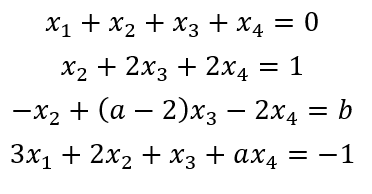当a和b分别取什么值时

1. 线性方程组有唯一解
2. 线性方程组无解
3. 线性方程组有无穷解，并求出通解。

解：

针对情况一：

线性方程组有唯一解的充要条件是R(A)=R(A,b)=n

Step1：这里我们构造增广矩阵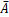=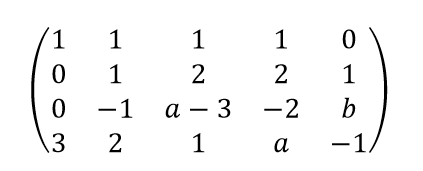Step2：第1行的-3倍加到第4行上去，则此时化为：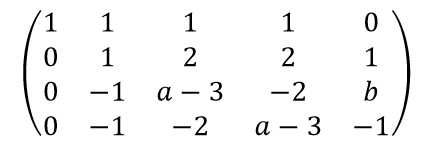Step3：针对step2，以第2行为轴，将第2行的1倍加到第3、4行上去，则化为：Step4：结合方程组唯一解条件，即R(A)=R(A,b)=n，这里n=4，那么比较容易得出a≠1时满足条件。即当a≠1时线性方程组有唯一解。

针对情况二：当R(A)<R(A,b)时无解，由Step3里化简后的阶梯矩阵可知

当a=1且b≠-1时R(A)=2,而R(A,b)=3即满足R(A)<R(A,b)。

所以a=1且b≠-1时线性方程组无解。

针对情况三：当R(A)=R(A,b)<n（n=4）时有无穷解。由Step3里化简后的阶梯矩阵可知

当a=1且b=-1时R(A)=2, R(A,b)=2，且都小于4，

所以当a=1且b=-1时线性方程组有无穷解。

关于通解：

对Step3里接着化简，即将第1列的-1倍加到第2、3、4列上去，则得到：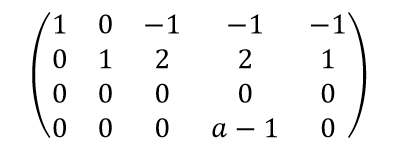那么我们容易得到原线性方程组等价于下式：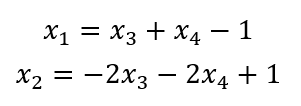那么该线性方程组的一般解

其中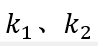为任意常数。

展开全文矩阵
• 当perC[G_c(A_1)]=,2,3,4时,本文给出了解线方程组AX=C的一种算法.G_c(A)是矩阵A的伴随有向图(Coates图),C[G_C(A)]是图G_C(A)的邻接矩阵.此算法将高斯消元过程直接在G_C(A)上进行,省去了化A为某种标准形的麻烦.此算法...
• Jacobi迭代法求解线性方程组以及Gauss-Seidel迭代法求解线性方程组的程序，C语言
• 研究生学习数值分析时一行一行写的，Guass列主元消元法求解线性方程组，找主元->换行->变A为上三角矩阵->回代求值。程序移植性强，注释详细，可以用来学习一下。
• 线性方程组 首先，我们来说一下定义： 线性方程：表示成a_1x_1+a_2x_2+a_3x_3+\cdots+a_nx_n=c\$的方程称为线性方程。 比如2×1−2=3×2,3×1+2−2×2=2×2−2+12x32x_1-2=3x_2,3x_1+2-2x_2=\sqrt2x_2-2+\dfrac12x_32...
• 利用Jacobi迭代法求解非线性方程组Ax=b,当系数矩阵A是严格对角占优矩阵或不可约对角占优时，该方法适用，里面附有详细注释，适合新手阅读Matlab
• 此函数计算方程组Ax=b的解，并将结果存放在数组b[0,n)中。 函数成功时返回false，否则返回true。 3. void qr(double* a, double* d, int n);矩阵的QR分解 假设数组anxn在内存中按行优先次序存放。此函数使用...
• 线性方程组的迭代法MATLAB源代码（共15个），具体函数及功能如下： 函数名 功能 rs 里查森迭代法求线性方程组Ax=b的解 crs 里查森参数迭代法求线性方程组Ax=b的解 grs 里查森迭代法求线性方程组Ax=b的解 jacobi ...迭代法
• 本文档利用Java编程语言求解线性方程组，不是原创，但很有用
• 使用 Gauss Seidel 方法求解线性方程组。 在这种情况下，A 是方程矩阵，向量 b 具有方程的右侧。matlab
• 线性方程组求解 1.直接法 Gauss 消元法 function x=DelGauss(a,b) % Gauss 消去法 [n,m]=size(a; nb=length(b; det=1% 存储行列式值 x=zeros(n,1; for k=1:n-1 for i=k+1:n if a(k,k)==0 return end m=a(i,k)/a(k,k;...
• 逐次超松弛迭代法解线性方程组Matlab程序 %---逐次超松弛迭代法----- %---successive over-reaxation iteration method clear;clc; A=[10,-1,-2;-1,10,-2;-1,-1,5]; b=[72,83,42]; N=length(b? %解向量的维数 ...
• 实验 用 matlab 求解线性方程组 第一节 线性方程组的求解 一齐次方程组的求解 rref A %将矩阵 A 化为阶梯形的最简式 nullA %求满足 AX0 的解空间的一组基即齐次线性方程组的基 础解系 例 求下列齐次线性方程组的一个...
• 采用递推最小二乘法求解超定线性方程组 Ax=b，其中 A 为 mxn 维的已知矩阵，b 为 m 维的已知向量，x 为 n 维的未知向量，其中 n=10，m=10000。A 与 b 中的元素服从独立同 分布的正态分布。绘出横坐标为迭代步数时的...
• 这个过程的第一步是将线性联立方程直接转换为矩阵形式，对于部分旋转，您需要手动输入方程式，如果方程式的第一个系数为零，则不能将方程式放在第一位。在这种情况下，我们需要在另一个方程之间交换。此代码的内循环...matlab
• 求上三角系数矩阵的线性方程组Ax=b的解，数值计算，求解方程
• 此函数将采用矩阵形式 Ax=b 的方程组，并使用雅可比迭代法计算变量 x=(x_1,x_2,...,x_n)。 为了确保收敛，使 A 矩阵对角占优。 该输出专用于3x3的A矩阵，但可以轻松省略或更改以适应您的需求。matlab
• 线性方程组求解A linear equation is an equation that graphs a line. A system of linear equations is when there are two or more linear equations grouped together. 线性方程是绘制直线的方程。 线性方程组是...

求解线性方程组

A linear equation is an equation that graphs a line. A system of linear equations is when there are two or more linear equations grouped together.

线性方程是绘制直线的方程。 线性方程组是当两个或多个线性方程组组合在一起时。

To simplify the illustration, we will consider systems of two equations. As the name suggests, there are two unknown variables. Often they are designated by the letters x and y. If equations describe some process, the letters can be chosen by the roles they play. For example, d can stand for distance, and t for time.

为了简化说明，我们将考虑两个方程式的系统。 顾名思义，有两个未知变量。 通常，它们由字母xy表示 。 如果方程式描述了某个过程，则可以通过字母扮演的角色来选择字母。 例如， d可以代表距离， t可以代表时间。

In this article we will learn how to solve systems of linear equations using two fun methods. But before we start, let's see how we end up with a particular system by looking at a real life example.

在本文中，我们将学习如何使用两种有趣的方法来求解线性方程组。 但是，在开始之前，我们先来看一个真实的例子，看看如何最终使用特定的系统。

## 派生系统 (Deriving a system)

A boy gets on his bicycle and starts riding to school. He rides 200 yards every minute.

一个男孩骑上自行车开始上学。 他每分钟骑200码。

6 minutes later, his mother realizes her son forgot his lunch. She gets on her own bicycle and starts following the boy. She rides 500 yards every minute (She is an Olympian and a gold medalist).

6分钟后，他的母亲意识到儿子忘记了午餐。 她骑着自己的自行车，开始跟随男孩。 她每分钟骑500码(她是奥运选手和金牌得主)。

We want to figure out how long it takes the mother to catch up to the boy, and how far she needs to ride to do so.

我们想弄清楚母亲要赶上男孩多长时间，以及她需要骑多远才能赶上男孩。

Since the boy covers 200 yards every minute, in t minutes he will cover 200 times t yards, or 200t yards.

由于男孩涵盖每分钟200码，在t分钟 ，他将覆盖200周 时间t码，或200吨码。

His mother starts bicycling 6 minutes later, so she rides for (t - 6) minutes. Since she covers 500 yards every minute, in (t - 6) minutes she covers 500 times (t - 6) yards, or 500(t - 6) yards.

他的母亲在6分钟后开始骑自行车，所以她骑了(t-6)分钟。 因为她每分钟覆盖500码，所以在(t-6)分钟内她覆盖了500(t-6)码，即500(t-6)码。

By the time she catches up to him, they both have covered the same distance. Let's say for now that distance is d.

当她赶上他时，他们俩已经跨越了相同的距离。 现在说距离是d

For the boy we have  d = 200t and for his mother we have d = 500(t - 6). We now have our system of two equations.

对于男孩，我们的d = 200t ，对于他母亲，我们的d = 500(t-6) 。 现在，我们有了两个方程式的系统。

A curly brace is often added to indicate that equations form a system.

通常添加大括号以指示方程式构成一个系统。

Now let's see how we can solve this system.

现在让我们看看如何解决这个系统。

## 替代解决 (Solving by substitution)

The first method we will consider uses substitution.

我们将考虑的第一种方法是使用替换

We have two unknowns here, d and t. The idea is to get rid of one variable by expressing it using the other variable.

我们在这里有两个未知数dt 。 这个想法是通过使用另一个变量来表达它来摆脱一个变量。

The top equation tells us that d = 200t, so let's plug in 200t for the d in the bottom equation. As a result, we have an equation with just the t variable.

顶部方程式告诉我们d = 200t ，因此让我们在底部方程式中为d插入200t 。 结果，我们有了一个仅包含t变量的方程。

First we expand the right side: 500(t -6) = 500t - 500*6 = 500t - 3000.

首先我们扩展右侧：500(t -6)= 500t-500 * 6 = 500t-3000

Then we simplify by moving the unknown members to one side and the known members to the other. The result is: 500t - 200t = 3000.

然后，我们将未知成员移到一侧，将已知成员移到另一侧，从而简化了操作。 结果是： 500t-200t = 3000

Solving for t gives us t = 10, or since we measure time in minutes, t = 10 minutes. In other words, the mother will catch up to her son in 10 minutes.

求解t得出t = 10 ，或者因为我们以分钟为单位测量时间，所以t = 10分钟 。 换句话说，母亲将在10分钟内赶上她的儿子。

The second part of our problem is to find out how far she had to cycle to catch up with him.

我们问题的第二部分是找出她必须骑自行车多远才能赶上他。

To answer that question, we need to find d. Substituting t = 10 in either equation will give us that answer.

要回答这个问题，我们需要找到d 。 将t = 10代入任一等式将得到答案。

To make it easier, let use the top equation, d = 200t = 200 * 10 = 2000. Since we measure distance in yards, d = 2000 yards.

为了简化起见 ，让我们使用顶部方程d = 200t = 200 * 10 = 2000 。 由于我们以码为单位测量距离，因此d = 2000码

Let's test your understanding so far – try to solve the next system on your own:

到目前为止，让我们测试您的理解–尝试自己解决下一个系统：

{
{

### y = 3(x-​​1) (y = 3(x - 1))

选择1个答案

In the system above, the unknown variables are x and y.

在上面的系统中，未知变量是xy

From the top equation we know that y = 2x. Substituting that to the bottom equation gives us 2(2x) = 3(x + 1).

从最上面的方程式我们知道y = 2x 。 将其代入底部方程式，我们得到2(2x)= 3(x + 1)

Once we expand and simplify, we get 4x = 3x + 3. Or x = 3. Therefore, y = 2 * 3 = 6.

扩展和简化后，我们得到4x = 3x + 3 。 或x = 3 。 因此， y = 2 * 3 = 6

## 通过图形求解 (Solving by graphing)

The second method we will consider uses graphing, where we find the solution to a system of equations by graphing them out.

我们将考虑的第二种方法是使用绘图 通过绘制方程式找到方程组的解。

For example, take this system: y = 2x + 3 and y = 9 - x.

例如，使用以下系统： y = 2x + 3y = 9-x

A graph of each equation will be a line. The first one for y = 2x + 3 looks like this:

每个方程的图形将是一条线。 y = 2x + 3的第一个看起来像这样：

Next, we can graph a line for y = 9 - x:

接下来，我们可以绘制一条y = 9-x的线

These two lines intersect at exactly one point. This point is the only solution to both equations:

这两条线恰好在一个点处相交 。 这是两个方程式的唯一解决方案：

The ordered pair (2, 7) gives us the coordinates of our point of intersection. This pair is the solution to the system. Substituting x = 2 and y = 7 will let us verify this.

有序对(2，7)为我们提供了相交点的坐标。 这对是系统的解决方案。 代入x = 2y = 7，我们将对此进行验证。

What if the graphs are parallel and do not intersect at all? For example:

如果图形是平行的并且根本不相交怎么办？ 例如：

When graphs of the equations do not intersect, that means our system has no solution. Trying to solve by substitution will prove that.

当方程的图不相交时，这意味着我们的系统没有解。 试图通过替代解决将证明这一点。

The result of x - 1 = x - 3 will be 0 = -2, which is always false.

x-1 = x-3的结果将为0 = -2 ，这始终为 false

But what if two graphs are the same and are directly on top of each other?

但是，如果两个图相同并且彼此直接位于顶部，该怎么办？

In such cases there are an infinite number of points of intersection. That means our system has an infinite number of solutions. Using the substitution method will prove that.

在这种情况下，交叉点的数量是无限的。 这意味着我们的系统拥有无限数量的解决方案。 使用替代方法将证明这一点。

The result of x - 2 = x - 2 is 0 = 0, which is always true.

x-2 = x-2的结果0 = 0 ，这始终是正确的

## 多练 (More practice)

Try using both the substitution and graphing methods to solve the following systems. These methods complement each other and will help you solidify your knowledge.

尝试同时使用替代方法和图形方法来解决以下系统。 这些方法相互补充，将帮助您巩固知识。

{
{

### 3y-2x = 4 (3y - 2x = 4)

选择1个答案

Choosing a particular variable to use in substitution should make finding a solution easier.

选择用于替换的特定变量将使查找解决方案变得容易。

Try expressing x with two other members in the top equation, then substitute the result into the bottom equation. That way you'll avoid dealing with fractions.

尝试用顶部方程式中的其他两个成员表示x ，然后将结果代入底部方程式。 这样，您将避免处理分数。

{
{

### 3x-2y = 4 (3x - 2y = 4)

选择1个答案

Let's do one more challenge:

让我们再做一个挑战：

{
{

### y = -x-1 (y = -x - 1)

选择1个答案

Now that you know enough about substitution and graphing, get out there and solve more linear equations.

既然您对替代和图形学已经足够了解，那么请走出去并解决更多的线性方程式。

求解线性方程组

展开全文java 人工智能 python 机器学习 算法
• 基于matlab求解线性方程组A*x=b，求解x向量，最终结果存储在x向量中。
• 平方根法(Cholesky分解法)解线性方程组 包括程序代码和结果分析 平方根法需要将矩阵做Cholesky分解，化为两个三角方程组求解。 % 平方根法(Cholesky分解法)解线性方程组Ax=b % A为方程组系数矩阵, b是方程组右端向量...
• ## matlab线性方程组求解

万次阅读 多人点赞 2020-08-21 15:42:31
线性方程组的求解主要有两种方法，分别是直接法和迭代法，本节也将围绕这两种方法去讲解一些matlab在求解线性方程组的相关知识。 一、线性方程组的直接解法 主要可以分为以下三种方法： 高斯（ Gauss ）消去法 ...

线性方程组的求解主要有两种方法，分别是直接法和迭代法，本节也将围绕这两种方法去讲解一些matlab在求解线性方程组的相关知识。
一、线性方程组的直接解法
主要可以分为以下三种方法：
高斯（ Gauss ）消去法
列主元消去法
矩阵的三角分解法
高斯（ Gauss ）消去法是一个经典的直接法，由它改进得到的列主元消去法，是目前计算机上求解线性方程组的标准算法，其特点就是通过消元将一般线性方程组的求解问题转化为三角方程组的求解问题。此外，还有矩阵的三角分解法等许多直接求解算法 。
1、利用左除运算符的直接解法
MATLAB 提供了一个左除运算符“\” 用于求解线性方程组，它使用列主元消去法，使用起来十分方便。对于线性方程组 Ax=b ，可以利用左除运算符反斜杠求解，b左除以A可获得线性方程组的数值解x。
注：这里的A和b是矩阵形式，且A里面的系数写法要与未知数对应。
例、用左除运算符求解下列线性方程组 。>>  A=[2,1,-5,1;1,-5,0,7;0,2,1,-1;1,6,-1,-4];
>> b=[13,-9,6,0]';
>>  x=A\b
x =
-66.5556
25.6667
-18.7778
26.5556


2、利用矩阵分解求解线性方程组
矩阵分解是设计算法的重要技巧，是指将一个给定的矩阵分解成若干个特殊类型矩阵的乘积 ，从而将一个一般的矩阵计算问题转化为几个易求的特殊矩阵的计算问题 。通过矩阵分解方法求解线性方程组的优点是运算速度快，可以节省存储空间 。
下面将主要去讲解矩阵分解中的LU分解：
（1） LU 分解的基本思想：
矩阵的 LU分解就是将一个n阶矩阵表示为一个下三角矩阵和一个上三角矩阵的乘积。线性代数中已经证明，只要方阵是非奇异的，LU 分解总是可以进行的。如下图对于三角方程很容易求解，于是可以首先求解向量y使 Ly=b，再求解 Ux =y，从而达到求解线性方程组 Ax=b 的目的。
（2）matlab的LU分解函数
LU 分解函数是根据列主元LU分解算法定义的，具有较好的数据稳定性。 lu函数有两种调用格式：
[L,U]= lu (A) ：产生一个上三角阵U和一个变换形式的下三角阵L，使之满足A=LU 。注意，这里的矩阵A必须是方阵。
[L,U,P]= lu (A) ：产生一个上三角阵U和一个下三角阵L以及一个置换矩阵P，使之满足 PA=LU 。同样，矩阵A必须是方阵。
注：当使用第一种格式时，矩阵L往往不是一个下三角阵，但可以通过行交换成为一个下三角阵。
还是以上述的线性方程组为例：

>> A=[2,1,-5,1;1,-5,0,7;0,2,1,-1;1,6,-1,-4];
>> b=[13,-9,6,0]';
>> [L,U]=lu(A);
>>  x=U\(L\b)
x =
-66.5556
25.6667
-18.7778
26.5556
>> [L,U,P]=lu(A);
>> x=U\(L\P*b)
x =
-66.5556
25.6667
-18.7778
26.5556


一、 线性方程组的迭代解法
迭代法是一种不断用变量的原值推出它的新值的过程，是用计算机解决问题的一种基本方法 。如下面图片所示介绍一种 线性方程组的迭代解法——雅可比（Jacobi）迭代法
对于线性方程组Ax=b，对于系数矩阵还是采取上述的矩阵分解的基本思想求解公式为：x(k+1)=D(-1)*（L+U）*x(k)+D(-1)*bmatlab中没有雅可比（Jacobi）迭代法的内置函数，因此此种方法需要自己编程实现，网上也有很多的代码，大家可以去了解一下，下面是网上的一段雅可比（Jacobi）迭代法的代码编写

function [y,n]=jacobi(A,b,x0,ep)
D=diag(diag(A));
L=-tril(A,-1);
U=-triu(A,1);
B=D\(L+U);
f=D\b;
y=B*x0+f;
n=1;
while norm(y-x0)>=ep
x0=y;
y=B*x0+f;
n=n+1;
end


其中，A是系数阵，b是方程组右边的常数，x0是迭代的初始值，ep是精度，在matlab 中将这段代码写入一个function，调用结果如下（以下面的方程组为例）:>> format long
>> A=[4,-2,-1;-2,4,3;-1,-3,3];
>> b=[1,5,0]';
>> [x,n]=jacobi(A,b,[0,0,0]',1.0e-6)
x =
0.970588483091126
0.852941039929380
1.176470992801838
n =
35
>> [L,U]=lu(A);
x=U\(L\b)

x =

0.970588235294118
0.852941176470588
1.176470588235294


可以看出两种方法算出来的结果，很是接近。
当然，除了此方法，还有很多的迭代方法去求解线性方程组，常见的有高斯- - 赛德尔（ Gauss- - Serdel ）迭代法，附上链接（https://blog.51cto.com/592669550/932010）
本节内容就到这里结束了，下节将推出非线性方程的求解，敬请期待！

关于MATLAB的学习：

大家可以关注我们的知乎专栏——数据可视化和数据分析中matlab的使用：
https://zhuanlan.zhihu.com/c_1131568134137692160

欢迎大家加入我们的MATLAB学习交流群：
953314432
扫码关注我们
发现更多精彩展开全文matlab
• 求解线性方程组 solvelinsolve 例 A=[5 0 4 2;1 -1 2 1;4 1 2 0;1 1 1 1]; %矩阵的行之间用分号隔开元素之间用逗号或空格 B=[3;1;1;0] X=zeros(4,1%建立一个 4 元列向量 X=linsolve(A,B) diff fun var n对表达式fun ......